# Online Electronics and Communication Engineering Test - Networks Analysis and Synthesis Test 3Loading Test...

Instruction:

• This is a FREE online test. DO NOT pay money to anyone to attend this test.
• Total number of questions : 20.
• Time alloted : 30 minutes.
• Each question carry 1 mark, no negative marks.
• DO NOT refresh the page.
• All the best :-).

1.

A network has two branches in parallel. One branch contains impedance Z1 and the other branch has impedance Z2. If it is fed from an ac voltage V of frequency f, the current through Z1 depends, on

A.
 V, Z1, Z2B.
 V, Z1C.
 Z1, Z2D.
 V, Z1, fExplanation:

In parallel branches, the current through any branch depends only on voltage, impedance and frequency only.

2.

In figure the voltage drop across the 10 Ω resistance is 10 V. The resistance R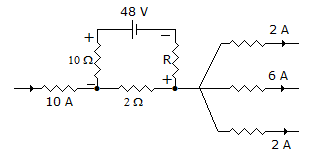A.
 is 6 ΩB.
 is 8 ΩC.
 is 4 ΩD.
 cannot be foundExplanation:

Current supplied by battery =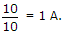Therefore current through 2Ω resistance is 11 A. Voltage across R = 48 - 10 - 11 x 2 = 16 V.

3.

Two similar coils have self inductance of 1 mH each. Coefficient of coupling is 0.5. The mutual inductance M is

A.
 0.25 mHB.
 0.5 mHC.
 0.707 mHD.
 1 mHExplanation:

M = kL1L2

4.

The rms voltage measured across each of the circuit element in figure is as shown, when the circuit is excited by a sinusoidal voltage Es, the rms value of the source voltage es is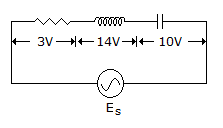A.
 10 voltB.
 2 voltC.
 EsD.
 5 voltExplanation:

Voltage across C = -10jv

Voltage across L = + 14 jv

source voltage Es = VR + jVL - jVC = 3 + j. 14 - j. 103 + j4

rms of Es = VR2(VL - VC)2 = 32 + 425 volt.

5.

The ABCD parameters of ideal n : 1 transformer shown in the figure are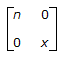. The value of X will be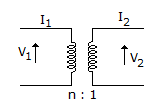A.
 nB.
 1/nC.
 n2D.
 1/n2Explanation: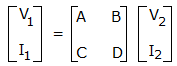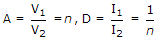.

6.

In figure V is ideal voltmeter having infinite resistance. It will read __________ volt.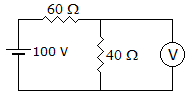A.
 40B.
 60C.
 0D.
 100Explanation: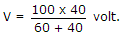7.

The correct relation between joules and calories is

A.
 calories = joules/4.156B.
 joules = calories/4.156C.
 calories = joules x 107/4.156D.
 joules = calories x 107/4.156Explanation:

Calories and joules are related by J, i.e., mechanical equivalent of heat.

8.

A certain network has two ideal voltages sources and a large number of ideal resistances. The power consumed in one of the resistances is 4 W when either of two sources is active and the other source is active and the other source is short circuited. When both sources is short circuited.When both sources are active, the power consumed in the same resistor would be

A.
 zero or 16 WB.
 4 W or 8 WC.
 zero or 8 WD.
 8 W or 16 WExplanation:

If currents of both sources add, total current would be twice and power is four time, i.e., 16 W. If currents subtract, net current and power are zero.

9.

Assertion (A): The impedance of a series resonant circuit is minimum at resonance.

Reason (R): Resonance condition implies unity power factor condition.

A.
 Both A and R are true and R is correct explanation of AB.
 Both A and R are true and R is not the correct explanation of AC.
 A is true but R is falseD.
 A is false but R is trueExplanation:

Since power factor is unity, impedance is minimum.

10.

The following circuit resonates at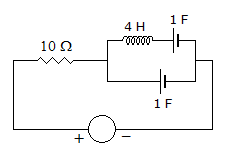A.
 0.5 HzB.
 5 HzC.
 1 rad/secD.
 0.5 rad/sec11.

A steel bar needs 1200 AT to magnetise it. The voltage that must be applied to the magnetising coil of 100 turns and 10 Ω resistance is __________ volt.

A.
 120B.
 12C.
 1200D.
 1012.

Poor power factor

A.
 reduces load handling capability of electrical systemB.
 results in more power losses in the electrical systemC.
 overloads alternators, transformers and distribution linesD.
 all13.

A reactance function is always a quotient of

A.
 even to odd polynomialsB.
 odd to even polynomialsC.
 even to odd or odd to even polynomialsD.
 even to even polynomials14.

The Z parameter of the following T-network is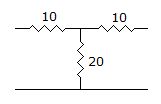A.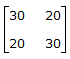B.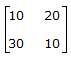C.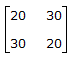D.
 not possible15.

In figure the effective resistance faced by voltage source is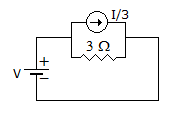A.
 1 ΩB.
 2 ΩC.
 3 ΩD.
 3.3 Ω16.

Which of the following statements is incorrect?

A.
 A mesh with two resistors has two mesh currentsB.
 The algebraic sum of all voltages around any mesh or any loop must be zeroC.
 The principle node is a function where branch current can divide or combineD.
 A Norton is a series circuit17.

In ZRC (s) = constant at s = 0, the last element in first Foster cauer realization is

A.
 RB.
 CC.
 R and C in seriesD.
 R and C in parallel18.

For a series circuit of R and variable XL the admittance locus is

A.
 a semicircle in first quadrantB.
 a semicircle in fourth quadrantC.
 a straight line in first quadrantD.
 a straight line in fourth quadrant19.

Assertion (A): In drawing an electric circuit analogous to a mechanical circuit, spring is replaced by capacitance.

Reason (R): The behaviour of a spring and capacitor are similar.

A.
 Both A and R are true and R is correct explanation of AB.
 Both A and R are true and R is not the correct explanation of AC.
 A is true but R is falseD.
 A is false but R is true20.

Norton's equivalent circuit

A.
 is same as Thevenin's circuitB.
 has no relation with Thevenin's circuitC.
 is dual of Thevenin's circuitD.
 is reciprocal of Thevenin's circuit